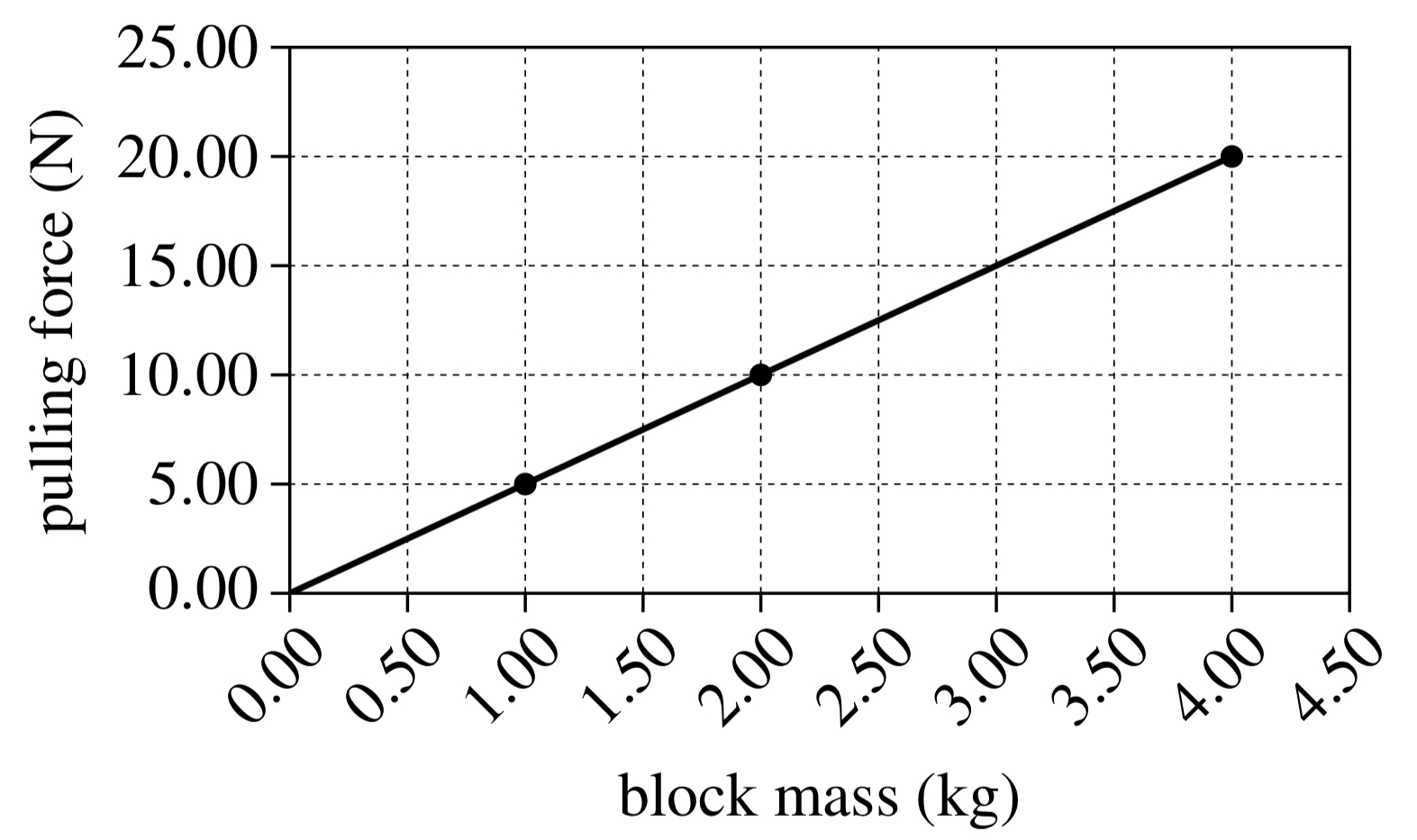ACT Energy
starstarstarstarstarstarstarstarstarstar
by Allison Van Liew
| 2 Questions
Note from the author:
Practice for ACT
In 2 experiments, a student pulled each of 3 blocks in a straight line across a flat, horizontal surface.

In Experiment 1, the student measured /pulling force / (the force required to move reach block at a constant speed) and plotted the pulling force in newtons (N), versus block mass, in kilograms (kg). The results are shown in Figure 1.In Experiment 2, the student measured the speed versus time of a 2.00 kg block, and a 3.00 kg block as each block wad puked across the surface with a constant 30 N force. The results are shown in Figure 2.1
1
If the block was pulled toward the east, the frictional force on the block by the surface was directed toward the
North
South
East
West
2
1
Based on Figure 2, what is the order of the 3 blocks, from the block that required the shortest time to reach 15 m/sec to the block that required the longest time to reach 15 m/sec?
2.00 kg block, 2.50 kg block, 3.00 kg block